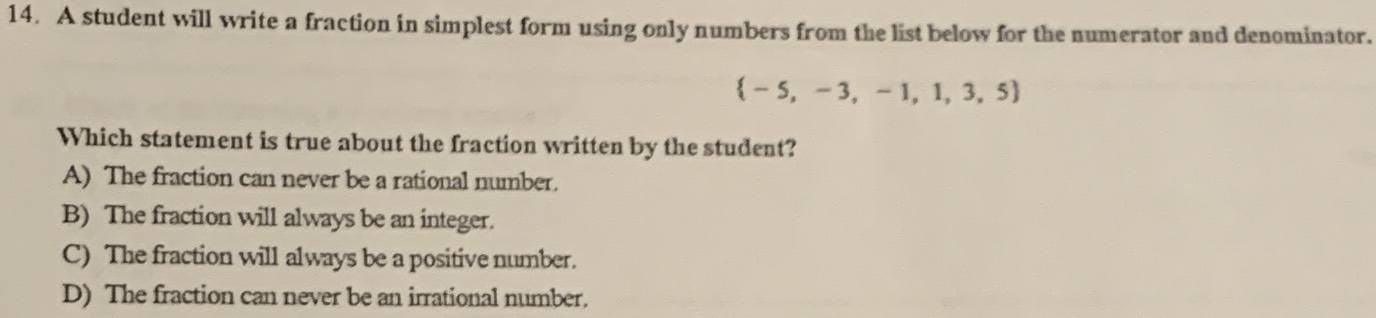### ¿Todavía tienes preguntas de matemáticas?

Pregunte a nuestros tutores expertos
Algebra
Pregunta14. A student will write a fraction in simplest form using only numbers from the list below for the numerator and denominator.

$$\{ - 5 , - 3 , - 1,1,3,5 \}$$

Which statement is true about the fraction written by the student?

A) The fraction can never be a rational number.

B) The fraction can never be a rational number.

C) The fraction will always be an integer.

D) The fraction can never be an irrational number.